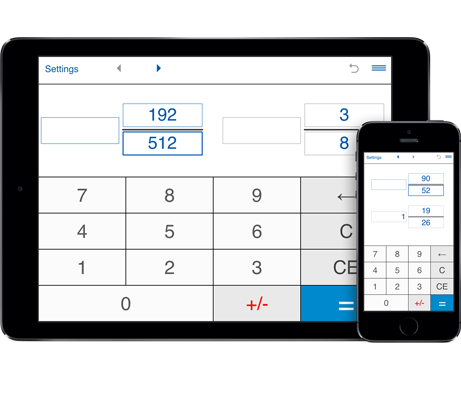# Simplify fractions calculator

A handy calculator that helps you simplify fractions step by step.
Features:
Supports proper, improper fractions and mixed numbers.
Prime factorization of the numerator and denominator.
Finds the Greatest Common Factor (GCF) of the numerator and denominator.
Reduces fractions to lowest terms.

## Simplifying fractions calculator - reduce fractions to lowest terms

Simplify fractions calculator helps you simplify fractions and mixed numbers.
To reduce fractions, enter the numerator and denominator (integer numbers only) and then press "=".
The calculator will find the Greatest Common Divisor (GCD) of the numerator and the denominator and then divide both the numerator and the denominator by their Greatest Common Factor (GCF). The calculator shows the fractions in their simplest form.
You may enter positive or negative numbers for both numerator and denominator.